Scattering and tunnelling

This free course is available to start right now. Review the full course description and key learning outcomes and create an account and enrol if you want a free statement of participation.

Free course

3.5 Scattering from finite square wells and barriers

The procedure used to analyse scattering from a finite square step can also be applied to scattering from finite square wells or barriers, or indeed to any combination of finite square steps, wells and barriers. The general procedure is as follows:

• Divide the x-axis into the minimum possible number of regions of constant potential energy.

• Write down the general solution of the relevant time-independent Schrödinger equation in each of these regions, remembering to use the appropriate value of the wave number k in each region and introducing arbitrary constants as necessary.

• Use continuity boundary conditions to determine all but one of the arbitrary constants. The one remaining constant is associated with the incident beam, which may enter from the right or the left.

• Obtain expressions for all the beam intensities relative to the intensity of the incident beam.

• Determine the reflection and transmission coefficients from ratios of beam intensities.

The best way to become familiar with this procedure is by means of examples and exercises. Below is a worked example involving a finite square well of the kind shown in Figure 12.

Worked Example 1

A particle of mass m with positive energy E0 is scattered by a one-dimensional finite square well of depth V0 and width L (shown in Figure 12). Derive an expression for the probability that the particle will be transmitted across the well.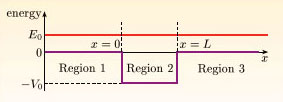Figure 12 A finite square well of depth V0 and width L. A beam of particles, each of energy E0, is scattered by the well

Solution

Suppose the particle concerned to be part of an intense steady beam of identical particles, each having energy E0 and each incident from the left on a well located between x = 0 and x = L.

Divide the x-axis into three regions: Region 1, (x < 0), where V = 0; Region 2, (0 ≤ xL), where V = −V0; and Region 3, (x > L), where V = 0. In each region the time-independent Schrödinger equation takes the general form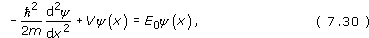so the solution in each region is: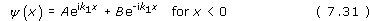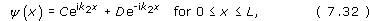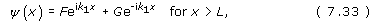where A, B, C, D, F and G are arbitrary constants, and the wave numbers in Region 1 and Region 2 are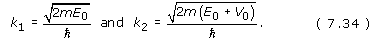Note that the wave number in Region 3 is the same as that in Region 1. This is because the potential energy function is the same in these two regions.

There is no leftward moving beam in Region 3, so we set G = 0. Since the potential energy function is finite everywhere,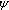(x) and d/dx must be continuous everywhere. Continuity of(x) and d/dx at x = 0 implies that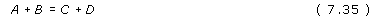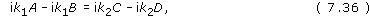while continuity of(x) and d/dx at x = L gives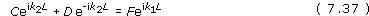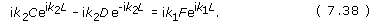Since the wave numbers in Regions 1 and 3 are both equal to k1, the intensities of the incident and transmitted beams are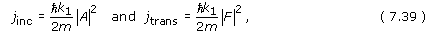and the required transmission coefficient is given by T = |F|2/|A|2.

The mathematical task is now to eliminate B, C and D from Equations 7.35 to 7.38 in order to find the ratio F/A. To achieve this, we note that the constant B only appears in the first two equations, so we take the opportunity of eliminating it immediately. Multiplying Equation 7.35 by ik1 and adding the result to Equation 7.36 we obtain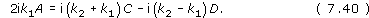Now we must eliminate C and D from the remaining equations. To eliminate D, we multiply Equation 7.37 by ik2 and add the result to Equation 7.38. This gives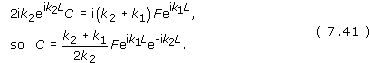Similarly, multiplying Equation 7.37 by ik2 and subtracting the result from Equation 7.38 we see that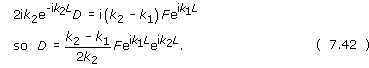Finally, substituting Equations 7.41 and 7.42 into Equation 7.40 and rearranging slightly we obtain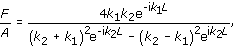so the transmission coefficient is given by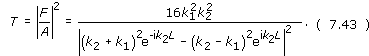This is the expression we have been seeking; it only involves k1, k2 and L, and both k1 and k2 can be written in terms of m, E0 and V0.

Although Equation 7.43 provides a complete answer to the worked example, it is expressed in a rather opaque form. After several pages of substitutions and manipulations (which are not a good investment of your time) it is possible to recast and simplify this formula. We just quote the final result: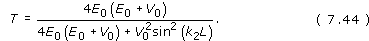Treating E0 as an independent variable and V0 as a given constant, this function can be displayed as a graph of T against E0/V0, as shown in Figure 13.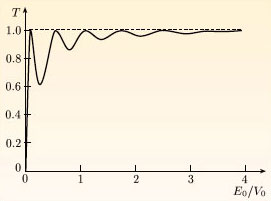Figure 13 The transmission coefficient T for a particle of energy E0 scattering from a finite square well of depth V0, plotted against E0/V0. To plot this graph, we have taken the particle to have the mass of an electron and taken the well to have a depth of 8.6 eV and a width of 1.0 nm

As the incident energy is increased significantly above 0 (the top of the well), transmission becomes relatively more likely, but there is generally some chance of reflection (R =1 − T ≠ 0), and for some energies the reflection probability may be quite high. However, Figure 13 also shows that there are some special incident particle energies at which T = 1, so that transmission is certain to occur. Although it is a rather poor use of terminology, these peaks of high transmission are usually called transmission resonances. Equation 7.44 shows that transmission resonances occur when sin(k2L) = 0, that is when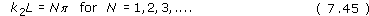Recalling the relationship k = 2/λ between wave number and wavelength, we can also express this condition as 2 = 2L; in other words:

A transmission resonance occurs when a whole number of wavelengths occupies the full path length 2L of a wave that crosses the width of the well and is reflected back again.

This condition can be interpreted in terms of interference between waves reflected at x = 0 and x = L. The interference turns out to be destructive because reflection at the x = 0 interface is accompanied by a phase change of. Of course, suppression of the reflected beam is accompanied by an enhancement of the transmitted beam. The effect is similar to one found in optics, where destructive interference between waves reflected from the front and back surfaces of a thin transparent film accounts for the success of the anti-reflective coatings on lenses and mirrors.

Before leaving the subject of scattering from a finite well there is one other point that deserves attention. This concerns the precise form of the functions(x) in the three regions we identified earlier. The value of |A|2 is equal to the linear number density of particles in the incident beam. If we regard A as being known, the values of the constants B, C, D and F can be evaluated using the continuity boundary conditions and graphs of(x) can be drawn.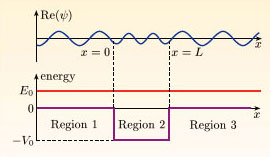Figure 14 A typical example of the real part Re(ψ) of the function ψ(x) for the kind of square well shown in Figure 12

Since(x) is generally complex there are several possible graphs that might be of interest, including the real part of, the imaginary part of, and ||2. Figure 14, above, shows a typical plot of the real part offor chosen values of m, E0, V0 and L; the following points should be noted:

1. In each region,(x) is a periodic function of x.

2. The wavelength is smaller inside the well than outside. The shorter wavelength corresponds to a higher wave number, and higher momentum, and echoes the classical increase in speed that a particle experiences as it enters the well.

3. The amplitude of the wave is smaller inside the well than outside. This is because the beam moves more rapidly inside the well, and has a lower linear number density there.

Exercise 8

With one modification, the stationary-state method that was applied to scattering from a finite square well can also be applied to scattering from a finite square barrier of height V0 and width L, when E0 > V0. Describe the modification required, draw a figure analogous to Figure 14, but for the case of a square barrier, and comment on any differences between the two graphs.

The main difference is that in the case of a finite square barrier V0 must be replaced by −V0 throughout the analysis. The wave number in Region 2 is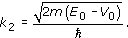Adapting Equation 7.44, the transmission coefficient is then given by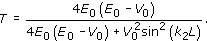A typical graph of the real part of(x) for scattering by a finite square barrier is shown in Figure 15, below. For a barrier, the wave number in Region 2 is decreased (corresponding to a reduced momentum eigenvalue) and the amplitude of the wave is generally greater in Region 2 than outside it (unless T = 1).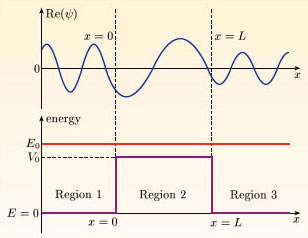Figure 15 A typical graph of the real part Re(ψ) of ψ(x) for a finite square barrier

Exercise 9

Draw two sketches to illustrate the form of the function |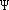(x, t)|2 in the case of the stationary-state approach to scattering from (a) a finite square well, and (b) a finite square barrier. Comment on the time-dependence of |(x,t)|2 in each case.

The graphs of |(x, t)|2 plotted against x for each case are shown in Figure 16.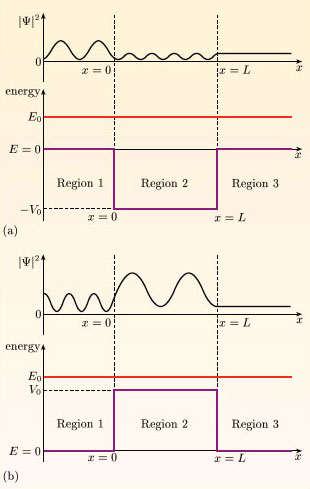Figure 16 |Ψ(x, t)|2 plotted against x for (a) a finite square well and (b) a finite square barrier

In Regions 1 and 2 interference effects lead to periodic spatial variations with a period related to the relevant wave number. In Region 3 there is only one beam, so there are no interference effects. Note that there is no simple relationship between the plots of |(x, t)|2 and the corresponding plots of the real part of(x, t). This is because |(x, t)|2 is partly determined by the imaginary part of(x,t). In both cases |(x, t)|2 is independent of time. This must be the case, despite the time-dependence of(x,t), because we are dealing with stationary states.

SM358_1